## Example Questions

← Previous 1

### Example Question #1 : How To Find Out If Lines Are Parallel

Which line below is parallel to  y – 2 = ¾x ?

y = 4/3 x + 5

y = ¾x - 5

y = 0

y = 2x + ¾

y = ¾x - 5

Explanation:

y – 2 = ¾x  is y = ¾x + 2 in slope intercept form (y=mx + b where m is the slope and b is the y-intercept). In this line, the slope is  ¾. Parallel lines have the same slope.

### Example Question #2 : How To Find Out If Lines Are Parallel

Assume line a and line b are parallel.

If angle x is three bigger than twice the square of four of angle y, then what is angle y?

7

5

10

8

6

7

Explanation:

Line a and b are parallel lines cut by a transverse line which make angle x and y alternate exterior angles. This means that angle x and angle y have the same measurement value.

The square root of 4 is 2; so twice 2 is 4. Then three added to 4 is 7. So x is equal to 7 and thus y is also equal to 7.

### Example Question #3 : How To Find Out If Lines Are Parallel

Two lines are described by the equations:

y = 3x + 5 and 5y – 25 = 15x

Which of the following is true about the equations for these two lines?

They represent parallel lines.

They represent non-perpendicular, intersecting lines.

They represent the same lines.

They represent perpendicular lines.

They represent the same lines.

Explanation:

The trick to questions like this is to get both equations into the slope-intercept form.  That is done for our first equation (y = 3x + 5).  However, for the second, some rearranging must be done:

5y – 25 = 15x; 5y = 15x + 25; y = 3x + 5

Note: Not only do these equations have the same slope (3), they are totally the same; therefore, they represent the same equation.

### Example Question #4 : How To Find Out If Lines Are Parallel

A line passes through the points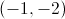and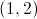. Which of the following lines is parallel to this line?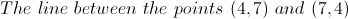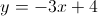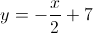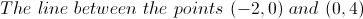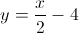Explanation:

Lines are parallel if they have the same slope. First, let's find the slope of the line betweenand.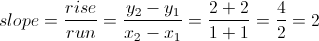So we are looking for a line with a slope of 2. We'll go through the answer choices.

The line between the points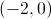and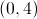: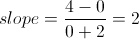. This is the same slope, so the lines are parallel, and this is the correct answer. We'll go through the rest of the answer choices for completeness.: This is in the form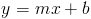, where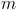is the slope. Here the slope is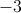, so this is incorrect.: Here the slope is, so this is again incorrect.: The slope is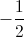, which is the negative reciprocal of 2. This line is perpendicular, not parallel, to the line in question.

The line between the points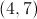and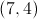: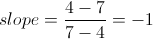, also incorrect.

### Example Question #1 : How To Find Out If Lines Are Parallel

All of the following systems of equations have exactly one point of intersection EXCEPT __________.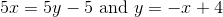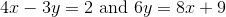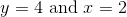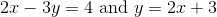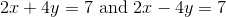Explanation:

In order for two lines to intersect exactly once, they can't be parallel; thus, their slopes cannot be equal. If two lines have slopes that are indeed equal, these lines are parallel. Parallel lines either overlap infinitely or they never meet. If they overlap, they intersect at infinitely many points (which is not the same as intersecting exactly once).

In other words, we are looking for the system of equations with lines that are parallel, because then they will either intersect infinitely many times, or not at all. If the lines are not parallel, they will intersect exactly once.

The only system of equations that consists of parallel lines is the one that consists of the lines 4x - 3y = 2 and 6y = 8x + 9. To determine whether or not these lines are parallel, we need to find their slopes. It helps to remember that the slope of a line in the standard form Ax + By = C is equal to -A/B. (Alternatively, you can solve for the slopes by rearranging both lines to slope-intercept form).

The line 4x - 3y = 2 is already in standard form, so its slope is -4/-3 = 4/3.

The line 6y = 8x + 9 is not in standard form, so we must rearrange it a little bit. First let's subtract 6y from both sides.

0 = 8x - 6y + 9

Then we can subtract 9 from both sides.

8x - 6y = -9

Now that the equation is in standard form, the slope is -8/-6 = 4/3.

Thus, these two lines are parallel, so they will either intersect infinitely many times, or not at all.

If we check all of the other systems of equations, we will find that each consists of lines that aren't parallel. Thus, all the other choices consist of lines that intersect exactly once.

The answer is the system of lines 4x - 3y = 2 and 6y = 8x + 9.

### Example Question #1 : How To Find Out If Lines Are Parallel

Line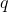is given by the equation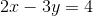. All of the following lines intersectEXCEPT: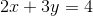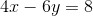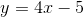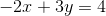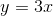Explanation:

In order for two lines to intersect, they cannot be parallel. Thus, we need to look at each of the choices and determine whether or not each line is parallel to line q, given by the equation 2x – 3y = 4.

To see whether or not two lines are parallel, we must compare their slopes. Two lines are parallel if and only if their slopes are equal. The line  2x – 3y = 4 is in standard form. In general, a line in the form Ax + By = C has a slope of –A/B; therefore, the slope of line q must be –2/–3 = 2/3.

Let's look at the line 2x + 3y = 4. This line is also in standard form, so its slope is –2/3. Because the slope of this line is not equal to the slope of line q, the two lines aren't parallel. That means line 2x + 3y = 4 will intersect q at some point (we don't need to determine where).

Next, let's examine the line y = 4x – 5. This line is in slope-intercept form. In general, a line in the form y = mx + b has a slope equal to m. Thus, the slope of this line equals 4. Because the slope of this line is not the same as the slope of q, these lines will intersect somewhere. We can eliminate y = 4x – 5 from our answer choices.

Similarly, y = 3x is in slope-intercept form, so its slope is 3, which doesn't equal the slope of q. We can eliminate y = 3x from our choices.

Next, let's analyze 4x – 6y = 8. The slope of this line is –4/–6 = 2/3, which is equal to the slope of q. Thus, this line is parallel to q. However, just because two lines are parallel doesn't mean they will never intersect. If two lines overlap, they are parallel, and they will intersect infinitely many times. In order to determine if 4x – 6y = 8 intersects line q, let's find a point on q and see if this point is also on the line 4x – 6y = 8.

Line q has the equation 2x – 3y = 4. When x = –1, y = –2. This means that q passes through the point (–1, –2). Let's see if the line 4x – 6y = 8 also passes through the point (–1, –2) by substituting –1 and –2 in or x and y.

4(–1) –6 (–2) = –4 + 12 = 8

The line 4x – 6y = 8 also passes through the point (–1, –2). This means that this line overlaps with line q, and they intersect infinitely many times.

By process of elimination, we are left with the line –2x + 3y = 4. However, let's verify that these lines don't intersect. The slope of this line is –(–2)/3 = 2/3, so that means it is parallel to line q. Let's see if this line passes through the point (–1, –2).

–2(–1) + 3(–2) = 2 – 6 = –4, which doesn't equal 4. In other words, this line doesn't pass through the same point as q. This means that the line –2x + 3y = 4 is parallel to q, but the two lines don't overlap, and thus can never intersect.

The answer is –2x + 3y = 4.

### Example Question #7 : How To Find Out If Lines Are Parallel

Which pair of linear equations represent parallel lines?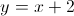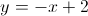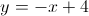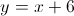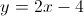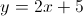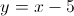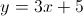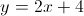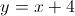Explanation:

Parallel lines will always have equal slopes. The slope can be found quickly by observing the equation in slope-intercept form and seeing which number falls in the "" spot in the linear equation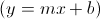We are looking for an answer choice in which both equations have the samevalue. Both lines in the correct answer have a slope of 2, therefore they are parallel.

### Example Question #8 : How To Find Out If Lines Are Parallel

Which of the following equations represents a line that is parallel to the line represented by the equation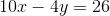?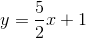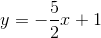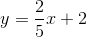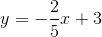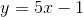Explanation:

Lines are parallel when their slopes are the same.

First, we need to place the given equation in the slope-intercept form.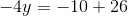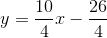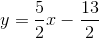Because the given line has the slope of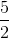, the line parallel to it must also have the same slope.

### Example Question #9 : How To Find Out If Lines Are Parallel

Which of the following lines is parallel to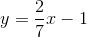?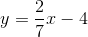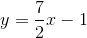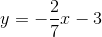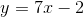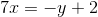Explanation:

The line presented in the question is. Parallel lines have the same slope. The slope of the presented equation is. Therefore, the answer is:### Example Question #10 : How To Find Out If Lines Are Parallel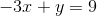is parallel to which of the following lines?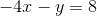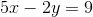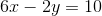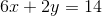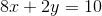Remember that when a line is stated as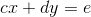, its slope-intercept conversionhas a slope of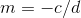.
Also bear in mind that we are looking for a parallel line, which means we need to find another line where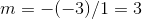.is our answer because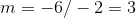, which is what we are looking for.